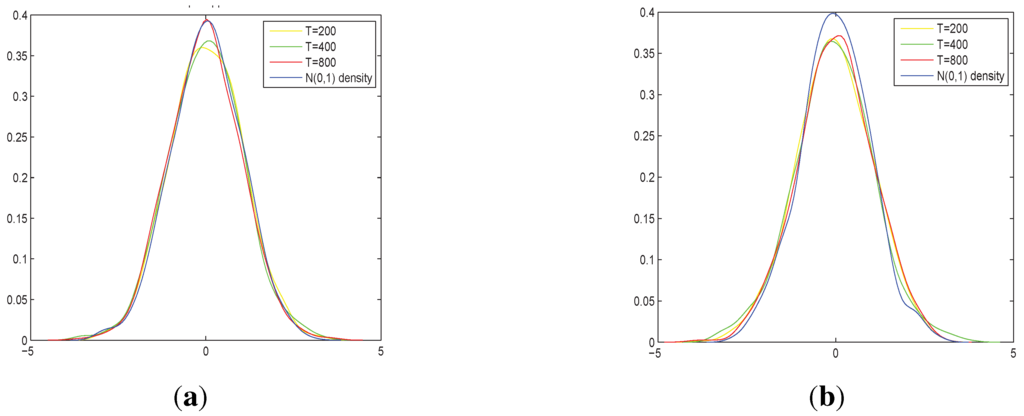Next Article in Journal
Selection Criteria in Regime Switching Conditional Volatility Models
Previous Article in Journal
Detecting Location Shifts during Model Selection by Step-Indicator Saturation
Previous Article in Special Issue
Finding Starting-Values for the Estimation of Vector STAR Models
Open AccessArticle

# Nonparametric Regression Estimation for Multivariate Null Recurrent Processes

byBiqing Cai andDag Tjøstheim *
Department of Mathematics, University of Bergen, 5020 Bergen, Norway
*
Author to whom correspondence should be addressed.
Econometrics 2015, 3(2), 265-288; https://doi.org/10.3390/econometrics3020265
Received: 26 November 2014 / Revised: 27 March 2015 / Accepted: 2 April 2015 / Published: 14 April 2015
This paper discusses nonparametric kernel regression with the regressor being a $$d$$-dimensional $$\beta$$-null recurrent process in presence of conditional heteroscedasticity. We show that the mean function estimator is consistent with convergence rate $$\sqrt{n(T)h^{d}}$$, where $$n(T)$$ is the number of regenerations for a $$\beta$$-null recurrent process and the limiting distribution (with proper normalization) is normal. Furthermore, we show that the two-step estimator for the volatility function is consistent. The finite sample performance of the estimate is quite reasonable when the leave-one-out cross validation method is used for bandwidth selection. We apply the proposed method to study the relationship of Federal funds rate with 3-month and 5-year T-bill rates and discover the existence of nonlinearity of the relationship. Furthermore, the in-sample and out-of-sample performance of the nonparametric model is far better than the linear model. View Full-Text
Show FiguresFigure 1

MDPI and ACS Style

Cai, B.; Tjøstheim, D. Nonparametric Regression Estimation for Multivariate Null Recurrent Processes. Econometrics 2015, 3, 265-288.